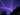# Storm Distance Calculator

## Distance

### Feet:   0

1. Click Start when you see the lightning
2. Click again when you hear the thunder

## How it Works

time x .21305 = distance

0 x .21305 = 0

Light travels so fast that a small calculator such as this would have a tough time using the speed of light in computations. So, the calculator assumes it's instant, when you see the lightning is the moment it happened. Click Start as close to seeing the lightning as possible. The closer the more accurate the calculation.

Once Start is clicked, the stopwatch starts counting the seconds until the thunder and Stop is clicked. Again, the closer to the thunder, the more accurate the distance calculation.

The speed of sound varies based on temperature, atmosphere, etc. So, we'll use 767 miles per hour, which equates to .21305 miles per second. Once we have a time in seconds, just multiply it by .21305 to get the distance.

You can always do something similar counting in your head. Once you see the lightning, start counting: one thousand one, one thousand two, one thousand three... The sound from the thunder travels one mile in about five seconds. So, take your count and divide by five to get the distance in miles.

Enjoy!